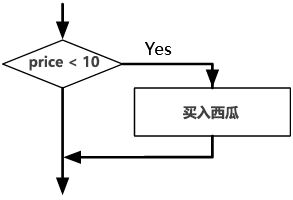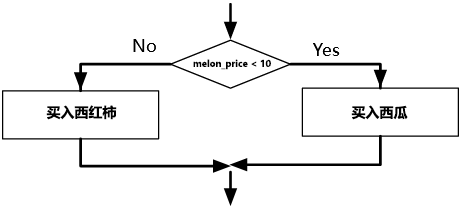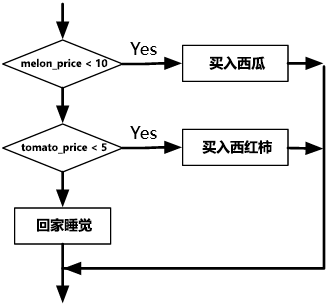# 条件执行语句

## 布尔表达式

``````print(5 == 5)
print(5 == 6)
``````
``````True
False
``````

``````print(type(True))
print(type(5 == 6))
``````
``````<class 'bool'>
<class 'bool'>
``````

``````x == y               # x 等于 y
x != y               # x 不等于 y
x > y                # x 大于 y
x < y                # x 小于 y
x >= y               # x 大于等于 y
x <= y               # x 小于等于 y
x is y               # x 与 y 是同一个对象
x is not y           # x 与 y 不是同一个对象
``````

## 逻辑运算

``````x and y     # x 和 y 必须都为 True 时结果为 True, 否则为 False
x or y      # x 或 y 存在任意一个为 True 则为 True， 否则为 False
not x       # x 为 True 则为 False， x 为 Flase 则为 True
``````
``````x > 2 and x < 5             # 当 x 大于2同时小于5时返回 True
x % 2 == 0 or x % 3 == 0    # 当 x 能被2或被3整除时返回 True
not (x > 2)                 # 当 x 不大于2时返回True
``````

## 条件执行

``````if price < 10:
print('买入西瓜')
``````

`if` 关键字后面的布尔表达式就被称为判断条件，我们的 `if` 判断条件语句以`:`（冒号）来结束。 下一行开始则为条件执行语句， 以缩进作为代码块的区分。``````if melon_price < 10:
print('买入西瓜')
else:
print('买入西红柿')
````````````if melon_price < 10:
print('买入西瓜')
elif tomato_price < 5:
print('买入西红柿')
else:
print('回家睡觉')
````````````if melon_price < 10:
print('买入西瓜')
elif tomato_price < 5:
print('买入西红柿')
if beer_price < pork_price:
print('回家炖西红柿牛肉')
else:
print('回家炖西红柿猪肉')
else:
print('回家睡觉')
``````

### 实战

1.引导用户输入整数，判断其是`偶数`还是`奇数`

2.同样是引导用户输入整数，判断其是否是`质数`

### 解析

1.引导用户输入整数，判断其是`偶数`还是`奇数`

``````while True:
inp = int(input('请输入整数：'))

if inp % 2 == 0:
print('你输入的是偶数')
else:
print('你输入的是质数')
``````
``````请输入整数：3

``````

2.同样是引导用户输入整数，判断其是否是`质数`

``````while True:
inp = int(input('请输入整数：'))

for n in range(2, inp):
if inp % n == 0:
print('你输入的不是质数')
break
else:
print('你输入的是质数')
``````
``````请输入整数：2

``````

å¿

2021-04-14

@å¿

2021-05-11

wwd

2021-06-24

• 1

• 1